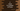# C program to check if a number is Pronic or not### C program to check if a number is Pronic or not :

In this tutorial, we will learn how to find a number is Pronic or not. A number is called a Pronic number if it is equal to the product of two consecutive numbers. For example, 7*8 = 56, so 56 is a Pronic number. In this program, we will ask the user to enter a number and then check if it is Pronic or not. Let’s take a look at the program :

### C program :

``````#include <stdio.h>

int main()
{
//1
int no;
int i;
int flag = 0;

//2
printf("Enter a number to check : ");
scanf("%d", &no);

//3
for (i = 0; i <= no; i++)
{ //4
if (i * (i + 1) > no)
{
break;
}

//5
if (i * (i + 1) == no)
{
printf("The input number is a Pronic number.\n");
flag = 1;
break;
}
}

//6
if (!flag)
{
printf("The input number is not a Pronic number.\n");
}
}``````

### Explanation :

The commented numbers in the above program denote the step number below :

1. Create one integer no to store the user-input number, integer i to use in the loop and one more integer flag to indicate if it is Pronic or not. flag = 0 means the number is not a Pronic number.
2. Ask the user to enter a number and store it in the variable no.
3. Run one for loop from 0 to the user input no.
4. Check if the product of current number and (current number + 1) is more than the user input no or not. If yes, break from the current loop because it cann’t be a Pronic number.
5. If the product of current number and (current no + 1) is equal to the number no, it is a Pronic number. Print one message , set the value of flag to 1 and break from the loop.
6. If the value of flag is still 0, print that the number is not a Pronic number.

### Sample Output :

``````Enter a number to check : 381
The input number is not a Pronic number.

Enter a number to check : 380
The input number is a Pronic number.

Enter a number to check : 0
The input number is a Pronic number.
``````